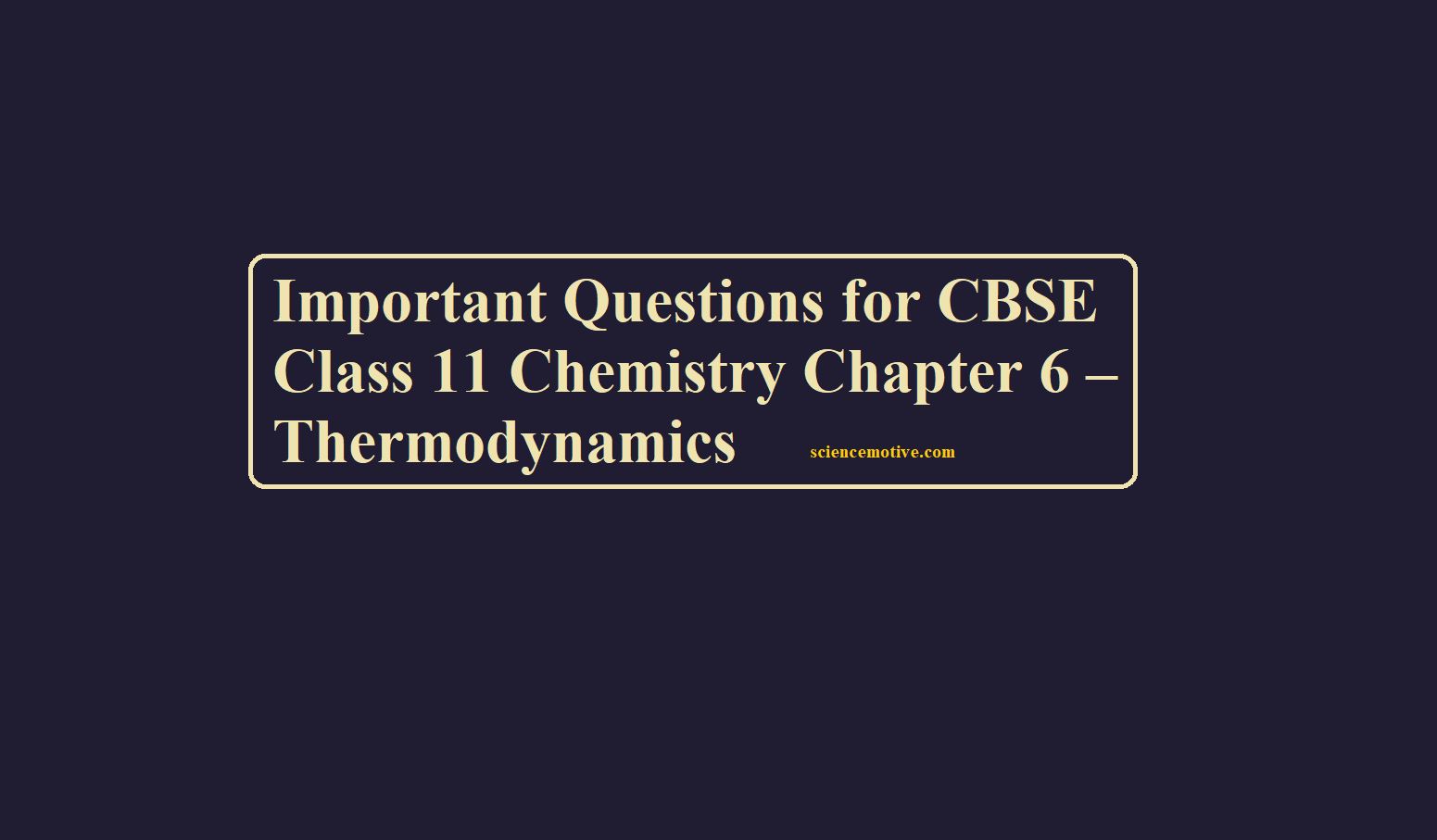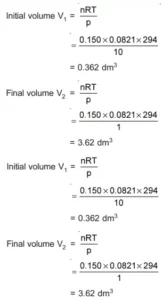# Important Questions for CBSE Class 11 Chemistry Chapter 6 – ThermodynamicsImportant Questions for CBSE Class 11 Chemistry Chapter 6 – Thermodynamics

Important Questions for CBSE Class 11 Chemistry

Que 1. What is meant by average bond energy? In what way is it different from the bond energy of a diatomic molecule? Give a suitable example.

Ans 1. Average bond energy is used in the case of polyatomic molecules because even though the molecule maybe contain identical bonds with identical bond length and energy, the energy required to break the individual bonds differ in each successive step. So, in such cases, average bond energy is used. For example, in CH4 molecule, all the four C–H bonds are equal in bond length and energy but differ in terms of energy required to break the bonds.
CH4(g) → C(g) + 4H(g); ΔaH0 = 1665 kJ mol–1
CH4(g) → CH3(g) + H(g); ΔbondH0 = + 427 kJ mol–1
CH3(g) → CH2(g) + H(g); ΔbondH0 = + 439 kJ mol–1
CH2(g) → CH(g) + H(g); ΔbondH0 = + 452 kJ mol–1
CH(g) → C(g) + H(g); ΔbondH0 = + 347 kJ mol–1
Therefore ΔaH0 = 427 + 439 + 452 + 347   = 416.25 kJ mol–1
4
It is different from the bond energy of a diatomic molecule because the energy required in diatomic molecules is to completely dissociate the molecules into atoms by breaking the covalent bonds of a gaseous covalent compound to form the products in the gaseous phase.

Que 2. (a) One mole of an ideal gas expands isothermally and reversible at 25ºC from a volume of 10 litres to a volume of 20 litres.
(i) What is the change in entropy of the gas?
(ii) How much work is done by the gas?
(iii) What is q(surroundings)?
(iv) What is the change in the entropy of the surroundings?
(v) What is the change in the entropy of the system plus the surroundings?
(b) Also answer the questions (i) to (v) if the expansion of the gas occurs irreversibly by simply opening a stopcock and allowing the gas to rush into an evacuated bulb of 10 L volume.
Ans 2. (i) ΔS = 2.303nR log (V2/V1) = 2.303 × 1 × 8.314 log (20/10) = 5.76 J/K.
(ii) wrev = – 2.303 nRT log (V2/V1) = – 2.303 × 1 × 298 × 8.314 log (20/10) = – 1718 J.
(iii) For isothermal process, ΔE = 0 and heat is absorbed by the gas,
qrev = ΔE – W = 0 – (–1718) = 1718 J
∴ qsurr = 1718 J. (∵ process is reversible).
(iv) ΔSsurr = – 1718/298 = –5.76 J/K. As entropy of the system increases by 5.76 J, the entropy of the surroundings decreases by 5.76 J, since the process is carried out reversible.
(v) ΔSsys + ΔSsurr = 0 ……. for reversible process.
(b) (i) ΔS = 5.76 J/K, which is the same as above because S is a state function.
(ii) w = 0. (∵ pext = 0)
(iii) No heat is exchanged with the surroundings.
(iv) ΔSsurr = 0
(v) The entropy of the system plus surroundings increases by 5.76 J/K, as we expect entropy to increase in an irreversible process.

Que 3. Justify the following statements.
(i) Reactions with ΔG0 < 0 always have an equilibrium constant greater than 1.
(ii) Many thermodynamically feasible reactions do not occur under ordinary conditions.
(iii) At low temperatures, enthalpy change dominates the expression ΔG and at high temperatures, it is the entropy that dominates the value of ΔG.
Ans 3. (i) ΔG = – 2.303 RT log K. Thus, when G0 < 0, K > 1.
(ii) Under ordinary conditions, the average energy of the reactants may be less than the threshold energy. They require some activations energy to initiate the reactions.
(iii) ΔG = ΔH -TΔS. At low temperatures, TΔS is small. Hence, ΔH dominates. At high temperature. TΔS is large, i.e., ΔS dominates the value of ΔG.

Que 4. Justify the following statements:
(i) An endothermic reaction is always thermodynamically spontaneous.
(ii) The entropy always increases on going from liquid to vapour state at any temperature T.
Ans 4. (a) It is false, exothermic reaction is not always spontaneous. If ΔS = +ve and TΔS > ΔH. The process will be non-spontaneous even it is endothermic.
(b) The entropy of vapour is more than that of liquid, so entropy increases during vaporization.

Que 5. A 0.150 mol sample of an ideal gas is allowed to expand at 294 K from 10.00 atm to 1.00 atm. If external pressure is kept constant at 1.00 atm. Calculate the work done.
Ans 5.Important Questions for CBSE Class 11 Chemistry